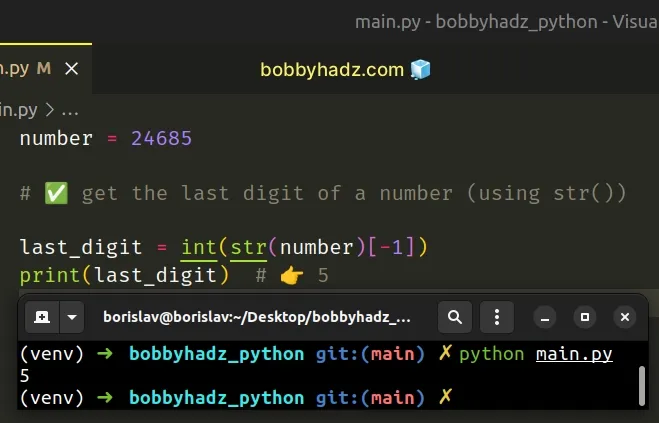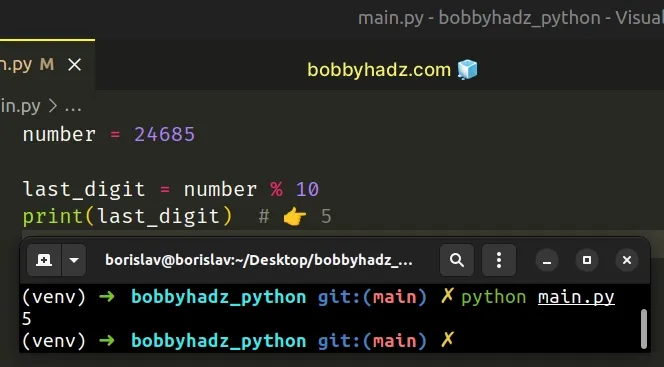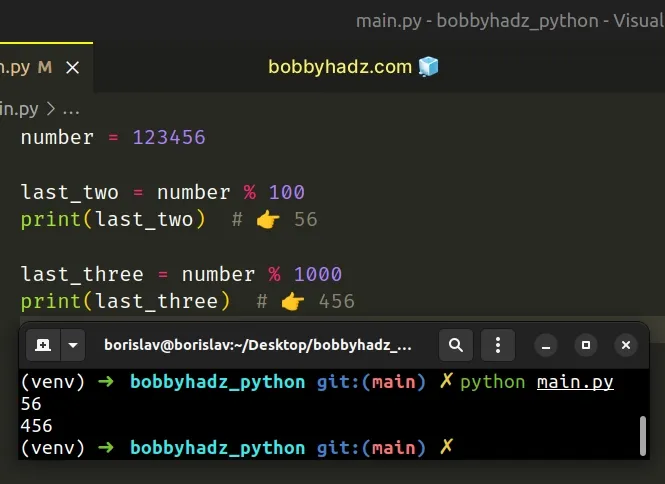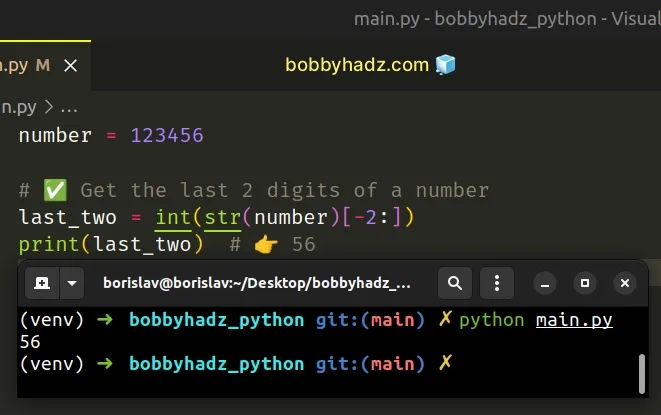# Get the last or last N digits of a Number in PythonLast updated: Feb 21, 2023
5 min## #Get the last digit of a number in Python

To get the last digit of a number:

1. Use the `str()` class to convert the number to a string.
2. Access the character at index `-1`.
3. Use the `int()` class to convert the result to an integer.
main.py
```Copied!```number = 24685

# ✅ get the last digit of a number (using str())

last_digit = int(str(number)[-1])
print(last_digit)  # 👉️ 5
``````If you need to get the last N digits of a number, click on the following subheading:

We used the str() class to convert the integer to a string so we can access the string at a specific index.

Python indexes are zero-based, so the first character in a string has an index of `0`, and the last character has an index of `-1` or `len(my_str) - 1`.

The next step is to access the character at index `-1` to get the last digit of the number.

Once we have the last digit, we can use the int() class to convert the string to an integer.

main.py
```Copied!```number = 24685

last_digit = int(str(number)[-1])
print(last_digit)  # 👉️ 5
``````

You can use the same approach if you need to get the last N digits of a number.

main.py
```Copied!```number = 24685

last_two = int(str(number)[-2:])
print(last_two)  # 👉️ 85

last_three = int(str(number)[-3:])
print(last_three)  # 👉️ 685
``````

Negative indices can be used to count backward.

## #Get the last digit of a number using the modulo operator

Alternatively, you can use the modulo operator.

main.py
```Copied!```number = 24685

last_digit = number % 10
print(last_digit)  # 👉️ 5
``````The modulo operator will return the last digit of the number by calculating the remainder of dividing the number by 10.

If the number might be negative, use the `abs()` function to make sure you get the correct result.

main.py
```Copied!```number = -24685

last_digit = abs(number) % 10
print(last_digit)  # 👉️ 5
``````

The abs function returns the absolute value of a number. In other words, if the number is positive, the number is returned, and if the number is negative, the negation of the number is returned.

main.py
```Copied!```print(abs(-27))  # 👉️ 27
print(abs(27))  # 👉️ 27
``````

The modulo (%) operator returns the remainder from the division of the first value by the second.

main.py
```Copied!```print(10 % 2)  # 👉️ 0
print(10 % 4)  # 👉️ 2
``````

If the value on the right-hand side is zero, the operator raises a `ZeroDivisionError` exception.

We used integers in the example, but the left and right-hand side values may also be floating point numbers.

The modulo `%` operator divides the number by `10` and returns the remainder.

For example, the remainder of dividing `12345` by `10` is `5`.

main.py
```Copied!```number = 12345

print(12345 - 1234 * 10)  # 👉️ 5
``````

Here are some more examples.

main.py
```Copied!```number = 12345

print(82342 % 10)  # 👉️ 2
print(248 % 10)  # 👉️ 8
print(150 % 10)  # 👉️ 0
``````

## #Get the last N digits of a Number in Python

You can also use the modulo operator to get the last N digits of a number.

The modulo operator in the example will return the last 2 digits of the number by calculating the remainder of dividing the number by 100.

main.py
```Copied!```number = 123456

last_two = number % 100
print(last_two)  # 👉️ 56

last_three = number % 1000
print(last_three)  # 👉️ 456
``````The same approach can be used to get the last 3 digits of a number.

main.py
```Copied!```number = 246810

# ✅ Get the last 3 digits of a number
last_three = number % 1000
print(last_three)  # 👉️ 810
``````

If the number might be negative, use the `abs()` function to make sure you get the correct result.

main.py
```Copied!```number = -123456

last_two = abs(number) % 100
print(last_two)  # 👉️ 56
``````

The abs function returns the absolute value of a number. In other words, if the number is positive, the number is returned, and if the number is negative, the negation of the number is returned.

main.py
```Copied!```print(abs(-43))  # 👉️ 43
print(abs(43))  # 👉️ 43
``````

The modulo (%) operator returns the remainder from the division of the first value by the second.

main.py
```Copied!```print(10 % 2)  # 👉️ 0
print(10 % 4)  # 👉️ 2
``````

If the value on the right-hand side is zero, the operator raises a `ZeroDivisionError` exception.

We used integers in the example, but the left and right-hand side values may also be floating-point numbers.

The modulo `%` operator divides the number by `100` and returns the remainder.

For example, the remainder of dividing `123456` by `100` is `56`.

main.py
```Copied!```number = 123456

print(123456 - 1234 * 100) # 👉️ 56
``````

Here are some more examples.

main.py
```Copied!```# ✅ Get the last 2 digits of a number
print(23484 % 100)  # 👉️ 84
print(9590 % 100)  # 👉️ 90
print(900 % 100)  # 👉️ 0
``````

And here is an example of getting the last three digits of a number.

The remainder of dividing `123456` by `1000` is `456`.

main.py
```Copied!```print(123456 - 123*1000)  # 👉️ 456
``````

Here are some more examples.

main.py
```Copied!```# ✅ Get the last 3 digits of a number
print(123456 % 1000)  # 👉️ 456
print(8523400 % 1000)  # 👉️ 400
print(1523000 % 1000)  # 👉️ 0
``````

Alternatively, you can use string slicing.

## #Get the last N digits of a Number using string slicing

This is a three-step process:

1. Use the `str()` class to convert the number to a string.
2. Use string slicing to get the last N characters of the string.
3. Use the `int()` class to convert the result to an integer.
main.py
```Copied!```number = 123456

# ✅ Get the last 2 digits of a number
last_two = int(str(number)[-2:])
print(last_two)  # 👉️ 56
``````The same approach can be used to get the last three digits of a number.

main.py
```Copied!```number = 246810

# ✅ Get the last 3 digits of a number
last_three = int(str(number)[-3:])
print(last_three)  # 👉️ 810
``````

We used the `str()` class to convert the integer to a string so we can use string slicing.

The syntax for string slicing is `my_str[start:stop:step]`.

The `start` index is inclusive, whereas the `stop` index is exclusive (up to, but not including).

Python indexes are zero-based, so the first character in a string has an index of `0`, and the last character has an index of `-1` or `len(my_str) - 1`.

Negative indices can be used to count backward.

The slice `my_str[-2:]` starts at the second to last character and goes to the end of the string.

Once we have the last 2 digits, we can use the `int()` class to convert the string to an integer.

main.py
```Copied!```number = 123456

last_two = int(str(number)[-2:])
print(last_two)  # 👉️ 56
``````

Which approach you pick is a matter of personal preference. I'd go with using the modulo `%` operator because it's quite intuitive and easy to read.